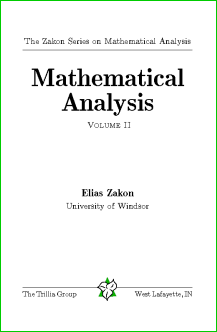Language/LinguaBooks 3054· Book News · Most clicked · Least clicked

Search for a Book## Mathematical Analysis IILanguage:Author: Elias ZakonFormat: PdfYear: 2009Category: AnalysisPages: 436Clicks: 671Description
This final text in the Zakon Series on Mathematics Analysis follows the release of the author's Basic Concepts of Mathematics and Mathematical Analysis I and completes the material on Real Analysis that is the foundation for later courses in functional analysis, harmonic analysis, probability theory, etc. The first chapter extends calculus to n-dimensional Euclidean space and, more generally, Banach spaces, covering the inverse function theorem, the implicit function theorem, Taylor expansions, etc. Some basic theorems in functional analysis, including the open mapping theorem and the Banach-Steinhaus uniform boundedness principle, are also proved. The text then moves to measure theory, with a complete discussion of outer measures, Lebesgue measure, Lebesgue-Stieltjes measures, and differentiation of set functions. The discussion of measurable functions and integration in the following chapter follows an innovative approach, carefully choosing one of the equivalent definitions of measurable functions that allows the most intuitive development of the material. Fubini's theorem, the Radon-Nikodym theorem, and the basic convergence theorems (Fatou's lemma, the monotone convergence theorem, dominated convergence theorem) are covered. Finally, a chapter relates antidifferentiation to Lebesgue theory, Cauchy integrals, and convergence of parametrized integrals. Nearly 500 exercises allow students to develop their skills in the area.

Similar Books
 Elements of Linear and Real Analysis Appunti di Analisi Matematica Mathematical Analysis I Semiclassical Analysis Theory of Functions of real variable Elementi della teoria delle funzioni analitiche Homeomorphisms in Analysis Why the Boundary of a Round Drop Becomes a Curve of Order Four Lectures on Entire Functions Constructive Real Numbers and Constructive Function Spaces Nonstandard Analysis Analytic functions Analyse II Analysis II Théorie élémentaire de l intégration de Henstock-Kurzweil General Topology and Real Analysis INTÉGRATION REAL ANALYSIS, SECOND EDITION ELEMENTARY REAL ANALYSIS Nonstandard Analysis LECTURES ON LIPSCHITZ ANALYSIS Nonstandard Analysis in Topology Introduction to real analysis Lectures on The Theory of Algebraic Functions of One Variable Analyse Réelle

```Home |  Authors | About | Contact Us |  Email